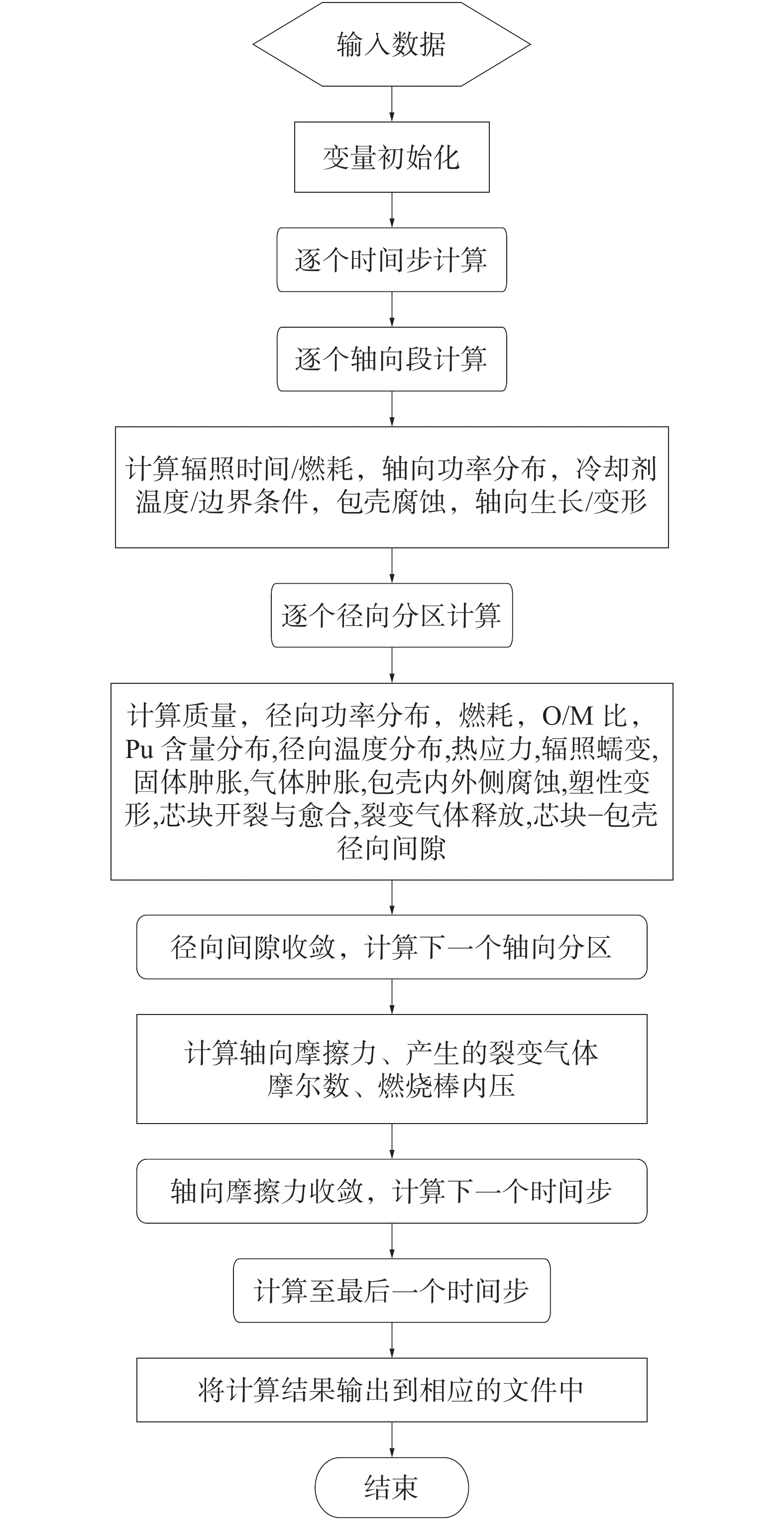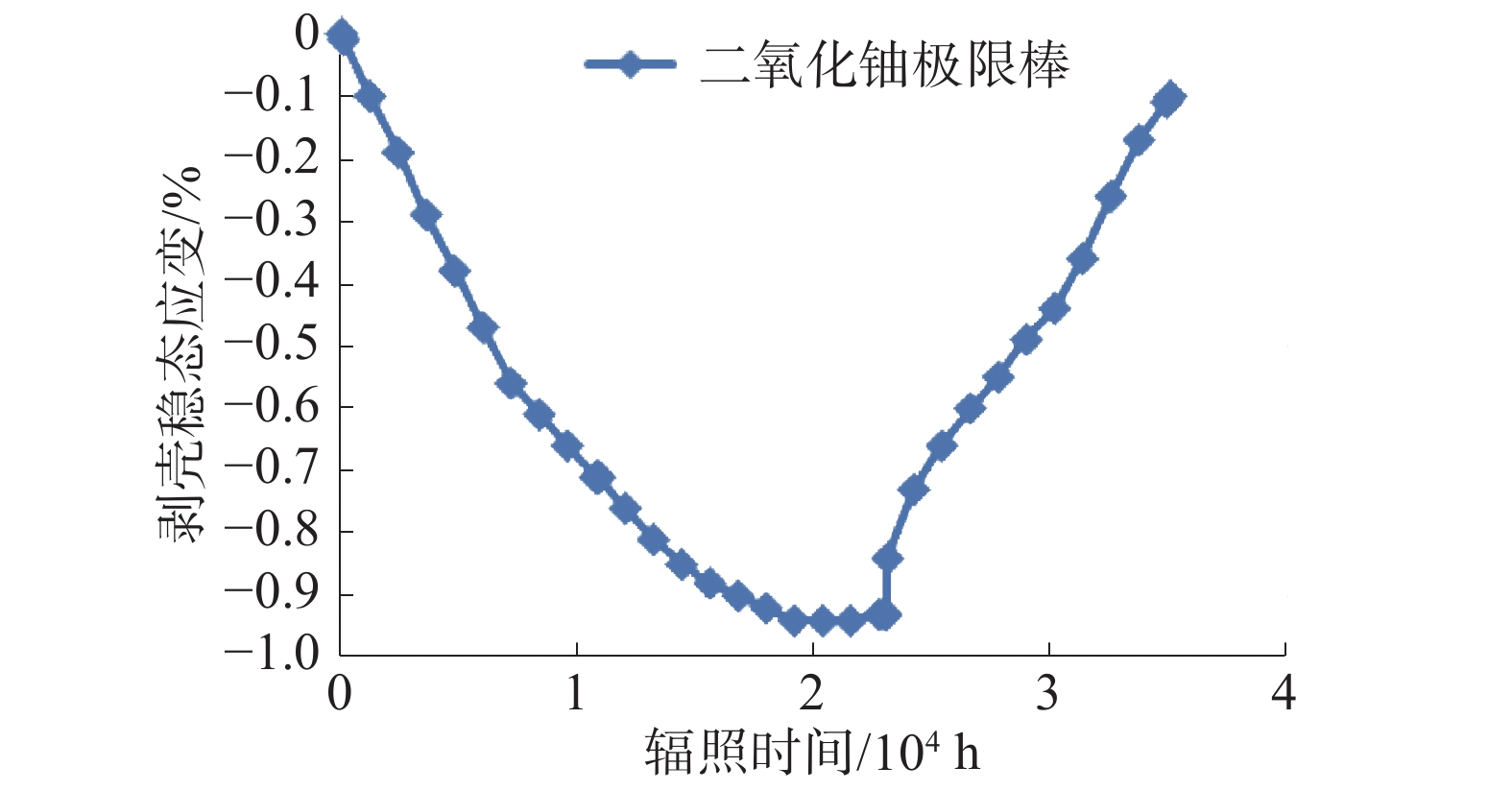﻿ 反应堆II类瞬态工况燃料棒包壳应变分析研究
«上一篇文章快速检索 高级检索

 应用科技2019, Vol. 46Issue (5): 76-79  DOI: 10.11991/yykj.2019030090

### 引用本文WANG Lu, PANG Hua, QING Tao, et al. Strain analysis of fuel rod cladding in reactor Class II transient conditions[J]. Applied Science and Technology, 2019, 46(5): 76-79. DOI: 10.11991/yykj.201903009.### 文章历史

Strain analysis of fuel rod cladding in reactor Class II transient conditions
WANG Lu, PANG Hua , QING Tao , ZHANG Kun , TANG Changbing , MIAO Yifei , YIN Mingyang
Science and Technology on Reactor Design Technology Laboratory, Nuclear Power Institute of China, Chengdu 610213, China
Abstract: In the new fuel design, in order to better ensure the integrity of the fuel rod, based on the background of long fuel cycle core fuel management for units 5 and 6 of Tianwan nuclear power plant, the FUPAC, a fuel performance analysis program independently developed by our institute, is used to simulate the mechanical behavior of core—cladding under steady state and Class II transient conditions, and the key parameters and models to be considered are studied, deriving the analytical method and theoretical model of reactor Class II transient cladding strain.
Keywords: cladding strain    long fuel cycle core    key parameters    transient    design criteria    mechanical behavior    fuel rod    fuel management

1 设计准则

2 计算模型

FUPAC软件可以模拟燃料棒在堆内辐照期间热/力学行为（包壳蠕变、辐照生长、腐蚀吸氢、芯块重定位、密实、肿胀和裂变气体释放等），计算热/力学参数（温度、应力、应变、氧化膜厚度、内压等）随辐照历史（反应堆运行工况、中子学数据）的变化。Download: 图 1 计算流程
2.1 芯块力学模型 2.1.1 气体肿胀模型

1）稳态气体肿胀模型

 $\Delta {V_{\rm{G}}} = {{\min}}[{f_1},\Delta {V_{\max}},{f_2}]$
 $\Delta {V_{\max}} = {\min}[{C_1},{C_2}{B_U}/{B_{{U_0}}}]$

2）瞬态气体肿胀模型

 $\begin{array}{c} \Delta {V_{\rm{G}}}\left( t \right) = \Delta {V_{\rm{G}}}\left( {{t_0}} \right) + \left[\Delta {V_{{\rm{GSS}}}}\left( t \right) - \Delta {V_{\rm{G}}}\left( {{t_0}} \right)\right] \times \\ {\rm{max}}\left[1,C{F_{{\rm{TR}}}}\left( t \right)/{F_{{\rm{SS}}}}\left( t \right)\right] \end{array}$

2.1.2 芯块密实化和固体肿胀模型

BU< ${B_{{U_{\lim }}}}$ 时：

 $\Delta \rho = 0$

BU $\geqslant{B_{{U_{\lim }}}}$ 时：

 $\Delta \rho = {\rho _0} \cdot a \cdot \ln \frac{{B_U}}{{20}} - {\rho _0} \cdot {k_0} \cdot a \cdot B_U$

2.1.3 芯块重定位应变模型

 ${e_{{\rm{relo}}}} = {e_{{\rm{sjort}}}} + {e_{{\rm{rearrangt}}}} + {e_{{\rm{crack}}}}$
 ${\varepsilon _{{\rm{relo}}}} = {\varepsilon _{{\rm{sjort}}}} + {\varepsilon _{{\rm{rearrangt}}}} + {\varepsilon _{{\rm{crack}}}}$

2.2 包壳力学模型详细说明 2.2.1 塑性应变模型

 ${\varepsilon _P} = {\left( {\frac{{\sigma - {\sigma _0}}}{K}} \right)^{1/n}}$

2.2.2 蠕变模型

2.2.3 包壳高应力蠕变和松弛模型

 $\varepsilon = {a_P} \cdot \ln (1 + {V_P} \cdot t) + {V_s} \cdot t$

3 验证实例

3.1 稳态应变Download: 图 2 包壳稳态应变随辐照时间的变化

3.2 瞬态应变

4 结论

1）分析得到影响包壳应变的主要影响因素，以及工程计算中需要考虑的不确定性因素；

2）通过自主化软件FUPAC程序验证计算，计算结果与技术限值相比较均有裕量，满足设计准则；

3）在新型燃料设计当中，需全面考虑引起燃料棒失效的因素以及计算模型带来的不确定性，在保证安全性的同时提高经济性。

  杨德锋, 肖小祥, 张晔, 等. 基于RELWWER程序的WWER型核电厂燃料棒破损分析[J]. 核安全, 2017, 16(3): 62-67. (0)  李田, 张学粮, 谢杰, 等. 压水堆核电站燃料组件的破损及管理策略[J]. 全面腐蚀控制, 2015(5): 80-83. (0)  司峰伟. 燃料组件破损原因分析及AP1000燃料的防护措施[J]. 科技创新与应用, 2013(25): 127. (0)  涂晓兰, 马永强, 冯晋涛. 燃料棒径向功率密度分布敏感性分析[J]. 科技视界, 2015(25): 283. DOI:10.3969/j.issn.2095-2457.2015.25.210 (0)  WANG Lianjie, ZHAO Wenbo, YANG Ping, et al. Development of SNTA code system for SCWR core steady-state analysis[J]. Journal of nuclear engineering and radiation science, 2017, 3(2): 021005. DOI:10.1115/1.4035334 (0)  黄海. UO2芯块热稳定性试验密度测定比对 [J]. 科技传播, 2016(2): 65-66. (0)  杨晓东. 含钆二氧化铀芯块制备及工业化应用研究[D]. 重庆: 重庆大学, 2008. (0)  李朝端. 气流粉碎技术在UO2粉末制造工艺中的应用研究 [J]. 原子能科学技术, 2001, 35(S1): 125-130. (0)  王雷, 屈平, 黄进浩, 等. 钛合金耐压结构蠕变数值计算方法与试验验证[J]. 船舶力学, 2019, 23(2): 190-199. DOI:10.3969/j.issn.1007-7294.2019.02.008 (0)  赵文金. M5合金的堆内外性能概述[J]. 核动力工程, 2001, 22(1): 60-64. DOI:10.3969/j.issn.0258-0926.2001.01.013 (0)  渠静雯, 王正品, 高巍, 等. M5锆合金马氏体热处理工艺研究[J]. 热加工工艺, 2013, 42(10): 172-174, 177. (0)  田锋, 李中奎, 张建军, 等. M5合金的高温低周疲劳性能研究[J]. 稀有金属, 2008, 32(6): 694-697. DOI:10.3969/j.issn.0258-7076.2008.06.004 (0)